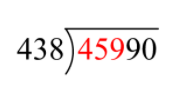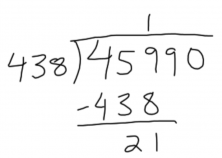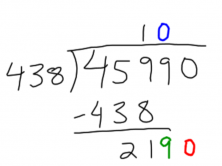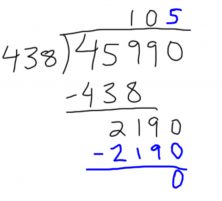Smartick is an advanced online program that teaches kids math and coding in only 15 min. a day

Jan18

# Learn How to Do a 3-Digit Division Problem

In this post, you are going to learn how to do 3-digit division. Before beginning to divide, it is important that you know the multiplication tables (1×1 through 9×9) because you need them to solve division.

Once you know the multiplication tables, you can begin to do 3-digit division. The steps that you must follow are:

1. Since the divisor has 3 digits, we must start with the first 3 digits of the dividend.
2. We compare the 3 digits of the dividend with the 3 digits of the divisor:
• If the number of the 3 digits of the dividend is greater than the number of the divisor, you can begin to divide.
• If the number of the 3 digits of the dividend is less than the number of the divisor, you have to start with the first 4 digits in the dividend.Since 459 is greater than 438, you can begin to do the division

3. Divide the digits of the dividend by the digits of the divisor.In order to divide 459 by 438, we take the first digit of each number and divide them: 4÷4 = 1

Write the 1 in the quotient and multiply it by the divisor: 438 x 1 = 438.

Now subtract: 459 – 438 = 21

4. Now bring down the next digit from the dividend and repeat the process.Divide 219 by 438. Since 438 goes into 219 zero times, put a 0 in the quotient and bring down another digit from the dividend.

Now divide 2190 by 438, which equals 5.The division ends when there are no more digits in the dividend to bring down.

Write 5 in the quotient and multiply by the divisor: 438 x 5 = 2190

Subtract: 2190 – 2190 = 0

Since we do not have more digits to bring down, we are finished with the division:

45990 ÷ 438 = 105; remainder = 0

This has been an example of 3-digit division, but you can find more examples and a more detailed explanation in this previous post:

If you liked this post, share it with your friends and colleagues so that they can also learn.

In order to not miss any updates from the Smartick blog,